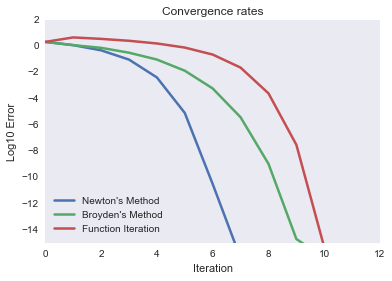# Convergence rates for different NLP methods¶

This demo shows how quickly different NLP methods converge to a solution. In particular, we look for the root of $$f(x) = \exp(x) - 1$$

starting with a guess $x_0 = 2$. The true solution is $x = 0$.

In :
from demos.setup import demo, np, plt
from compecon import NLP
%matplotlib inline


### Define a NLP problem¶

Here, we set convergence tolerance tol=1e-20 and the option all_x=True to record all values taken by $x$ from the initial guess x0=2.0 to the final solution. These values will be stored in the .x_sequence attribute.

We also define err to compute the base-10 logarithm of the error (the gap between the current iteration and the solution).

In :
A = NLP(lambda x: (np.exp(x)-1, np.exp(x)), all_x=True, tol=1e-20)
err = lambda z: np.log10(np.abs(z)).flatten()
x0 = 2.0


### Solve the problem¶

#### * Using Newton's method¶

In :
A.newton(x0)
err_newton = err(A.x_sequence)


#### * Using Broyden's method¶

In :
A.broyden(x0)
err_broyden = err(A.x_sequence)


#### * Using function iteration¶

This method finds a zero of $f(x)$ by looking for a fixpoint of $g(x) = x-f(x)$.

In :
A.funcit(x0)
err_funcit = err(A.x_sequence)


### Plot results¶

In :
demo.figure('Convergence rates', 'Iteration', 'Log10 Error',
[0, 12], [-15, 2])
plt.plot(err_newton, label="Newton's Method")
plt.plot(err_broyden, label="Broyden's Method")
plt.plot(err_funcit, label="Function Iteration")
plt.legend(loc='lower left')
plt.show()